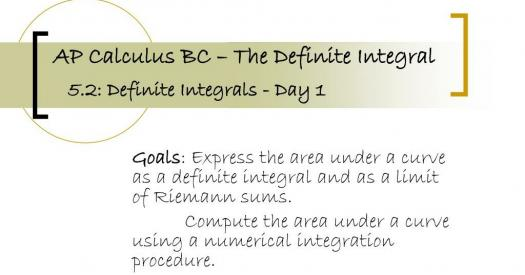# AP Calculus Integrals Practice Test!

10 Questions | Total Attempts: 207SettingsAP calculus is an integral part of high school and college mathematics. Engineering students and students of physics and chemistry also use it in solving complex problems. This quiz is built to help you understand calculus better and test your knowledge so far. Find a good desk and dig in!

• 1.
If 1/8 + 1/10 = v/y, and v and y are positive integers and v/y is in its simplest reduced form, what is the value of v?
• A.

9

• B.

18

• C.

27

• D.

36

• 2.
What is the differential coefficient of y = 2x/4 + x?
• A.

2/ (8 + x)²

• B.

4/ (1 - x)³

• C.

8/ (4 + x)²

• D.

8/ 4 + x²

• 3.
The product of integers x and y is divisible by 36. If x is divisible by 6, which of the following must be true? i. y is divisible by x ii. y is divisible by 6 iii. y/6 is divisible by 6
• A.

None

• B.

Ii only

• C.

I and iii only

• D.

Iii only

• 4.
Find dy/dx of sinX + x / x² + cosX
• A.

X - sinX + cosX + √x + 3√x / x²- cosX

• B.

X²- cosX + 1/2x + 3/ 2√x/ (2x²- sinX)

• C.

X³ - sinX - √2x - cos X / (x² + cosX)²

• D.

(x² + 1) cosX - XsinX - x² + 1 / (x² + cosX)

• 5.
If y = 3x² – In5x then d²y/dx² is equal to:
• A.

6 + 1/5x²

• B.

6x – 1/x

• C.

6 – 1/5x

• D.

6 + 1/x²

• 6.
A solution of the differential equation 3x²y dy/dx = y² – 3 given that x = 1 when y = 2 is:
• A.

3In( y² – 3) = In x² – In 4

• B.

Y = y³/3 – 3/x³y + 13/6

• C.

3/2 In (y² – 3) = 1- 1/x

• D.

2x² = y² – 6In x – 2

• 7.
Differentiating y = 1/√x + 2 with respect to x gives:
• A.

1√x³ + 2

• B.

− 1/2√x³

• C.

2 - 1/2√x³

• D.

2/√x³

• 8.
Differentiating  y= 4x5 gives:
• A.

Dy/dx = 2/3 x​​​​​​6

• B.

Dy/dx = 20x⁴

• C.

Dy/dx = 4x6

• D.

Dy/dx = 5x⁴

• 9.
Given f(t) = 3t⁴ − 2, f(t) is equal to:
• A.

12t³ − 2

• B.

3/4 t​​​​​​​​​​​​5 − 2t + c

• C.

12t³

• D.

3t5− 2

• 10.
If f(2) = 10 and f(4) = 44, which of the following could be f(x)?
• A.

2x + 6

• B.

2x2 + 12

• C.

3x2 - x

• D.

X - 6x

Related TopicsBack to top